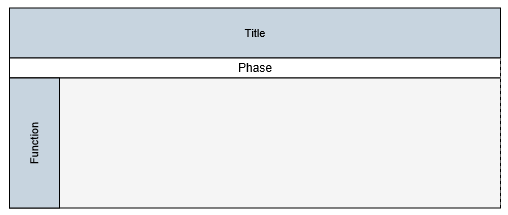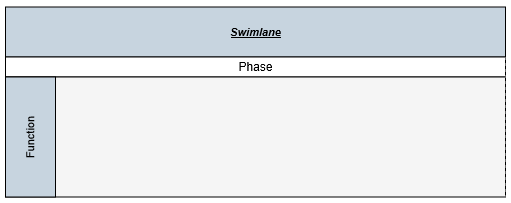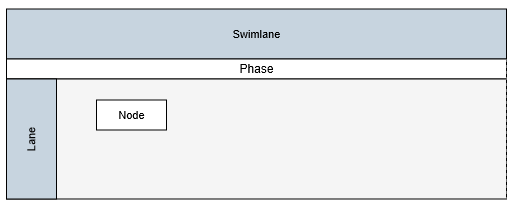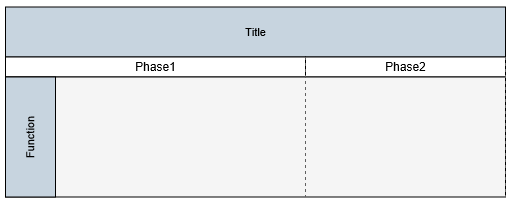# Swim lane

14 Oct 201612 minutes to read

Swim-lane Diagrams are typically used to visualize the relationship between a business process and the department responsible for it by focusing on the logical relationships between activities. Swimlanes may be arranged either horizontally or vertically.

##Initialize the Diagram model
Create a Object for diagram properties model element that can be used to initialize the diagram widget as shown below.

• RAZOR
• ``````<div>
<ej-diagram id="diagram" width="900px" height="600px"></ej-diagram>
</div>``````

## Create a swimlane

To create a swimlane, you need to use the `Swimlane` class. By default, the swimlanes are arranged vertically. You can change that with the `Orientation` property of swimlane.

The following code example illustrates how to define a swimlane object.

• RAZOR
• ``````<e-swim-lane name="SwimlaneNode" orientation="horizontal" offset-x="400" offset-y="200" width="700" height="100">
</e-swim-lane>``````

Adding a swimlane to the Diagram is same as adding a node. You can add either through the `Nodes` collection or through the client side method `add`. You can also drag and drop a swimlane from symbol palette.

The following code example illustrates how to add a swimlane to the Diagram through `Nodes` collection.

• RAZOR
• ``````<ej-diagram id="diagram" width="900px" height="600px">
<e-nodes>
<e-swim-lane name="SwimlaneNode" orientation="horizontal" offset-x="400" offset-y="200" width="700" height="100">
</e-swim-lane>
</e-nodes>
</ej-diagram>``````Swimlane allows to define a header to textually describe it. The `Header` property of swimlane allows you to define its textual description(`Text`) and to customize its appearance. The following code example illustrates how to define swimlane header.

• RAZOR
• ``````<ej-diagram id="diagram" width="900px" height="600px">
<e-nodes>
<e-swim-lane name="SwimlaneNode" orientation="horizontal" offset-x="400" offset-y="200" width="700" height="100">
</e-swim-lane>
</e-nodes>
</ej-diagram>``````
• JS
• ``````//Updates the swimlane header at runtime
var diagram = \$("#diagram").ejDiagram("instance");Swimlane headers can be updated at runtime with the client side method `updateNode`. The following code example illustrates how to update a lane’s header at runtime.

• JS
• ``````var diagram = \$("#diagram").ejDiagram("instance");

//Defines the header and format its text
text: "swimlane",
bold: true,
italic: true
};

You can hide the swimlane headers. The following code example illustrates how to hide headers.

• RAZOR
• ``<e-swim-lane-header text="Swimlane" height="0" fill-color="#C7D4DF" font-color="black" font-size="11" font-family="Arial" italic="true" bold="true" text-decoration="Underline"></e-swim-lane-header>``

## Lane

Lane is a functional unit or a responsible department of a business process that helps to map a process within the functional unit or in between other functional units.
You can add any number of lanes to a swimlane and the lanes are automatically stacked inside a swimlane based in the order they are added.

### Create an empty lane

To create an empty lane, you need to define an object with `IsLane` property that helps identify the object as a lane. The following example illustrates how to define a swimlane with a lane.

• RAZOR
• ``````<ej-diagram id="diagram" width="900px" height="600px">
<e-nodes>
<e-swim-lane name="SwimlaneNode" orientation="horizontal" offset-x="400" offset-y="200" width="700" height="100">
<e-lanes>
<e-lane name="lane1" fill-color="#f5f5f5" min-height="120"></e-lane>
</e-lanes>
</e-swim-lane>
</e-nodes>
</ej-diagram>``````

### Create a lane with header

The `Header` property of the lane allows you to textually describe the lane(`text`) and to customize the appearance of the description. The following code example illustrates how to define a lane header.
You can limit the size of a lane with its `MinWidth`, `MinHeight`, `MaxWidth`, and `MaxHeight` properties.

• RAZOR
• ``````<ej-diagram id="diagram" width="900px" height="600px">
<e-nodes>
<e-swim-lane name="SwimlaneNode" orientation="horizontal" offset-x="400" offset-y="200" width="700" height="100">
<e-lanes>
<e-lane name="lane1" fill-color="#f5f5f5" height="120" min-height="120" min-width="500" max-height="200" max-width="700">
</e-lane>
</e-lanes>
</e-swim-lane>
</e-nodes>
</ej-diagram>``````

You can disable/update the lane header at runtime with the client side method, `updateNode`. The following code example illustrates how to disable the lane header at run time.

• JS
• ``````var diagram = \$("#diagram").ejDiagram("instance");

diagram.updateNode("laneName", { header: {height: 0} })``````

### Add nodes to a lane

To add nodes to a lane, You need to add them to the `Children` collection of lane. The following code example illustrates how to add nodes to a lane.

• RAZOR
• ``````<ej-diagram id="diagram" width="900px" height="600px">
<e-nodes>
<e-swim-lane name="SwimlaneNode" orientation="horizontal" offset-x="400" offset-y="200" width="700" height="100">
<e-lanes>
<e-lane name="lane1" fill-color="#f5f5f5" height="120" min-height="120" min-width="500" max-height="200" max-width="700">
<e-children>
<e-node name="child" width="70" height="30" margin-left="70" margin-top="1">
<e-labels>
<e-diagram-label text="Node"></e-diagram-label>
</e-labels>
</e-node>
</e-children>
</e-lane>
</e-lanes>
</e-swim-lane>
</e-nodes>
</ej-diagram>``````## Phase

Phases are the sub-processes that are used to break the swimlane into multiple smaller regions.

To define a phase, you have to set the length of the region to the `Offset` property of phase. Every region can be textually described with the `Label` property of phase.

The following code example illustrates how to add a phase on initializing swimlane.

• RAZOR
• ``````<ej-diagram id="diagram" width="900px" height="600px">
<e-nodes>
<e-swim-lane name="SwimlaneNode" orientation="horizontal" offset-x="400" offset-y="200" width="500" height="100">
<e-lanes>
<e-lane name="lane1" fill-color="#f5f5f5" height="120" min-height="120">
</e-lane>
</e-lanes>
<e-phases>
<e-phase name="Phase1" offset="300" line-width="1" line-dash-array="3 3" line-color="#606060">
<e-label text="Phase1"></e-label>
</e-phase>
<e-phase name="Phase2" line-width="1" line-dash-array="3 3" line-color="#606060">
<e-label text="Phase2"></e-label>
</e-phase>
</e-phases>
</e-swim-lane>
</e-nodes>
</ej-diagram>``````You can add a region at runtime with the client side method, `addPhase`. The following code example illustrates how to add a phase at runtime.

• JS
• ``````var phase = {
name: "Phase3",
label: { text: "Phase3" }
};

var diagram = \$("#diagram").ejDiagram("instance");

A phase can be updated at runtime with the client side API `updateNode`. The following code example illustrates how to a update phase at runtime.

• JS
• ``````var diagram = \$("#diagram").ejDiagram("instance");
var options = {
//Specifies the style of the phase to be updated
lineDashArray: "3,3",
lineColor: "#C7D4DF",
lineWidth: 2
}
diagram.updateNode("phaseName", options);``````

NOTE

A default phase is added, when the phase collection of the swimlane is empty. When the phase collection is initialized, a default phase is appended at the end of swimlane.

## Limitations

• You cannot add connectors as the children of lanes.